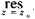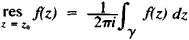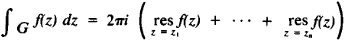# residue

(redirected from cannery residue)
Also found in: Dictionary, Thesaurus, Medical, Legal.

## residue

Law what is left of an estate after the discharge of debts and distribution of specific gifts
Collins Discovery Encyclopedia, 1st edition © HarperCollins Publishers 2005
The following article is from The Great Soviet Encyclopedia (1979). It might be outdated or ideologically biased.

## Residue

(1) In the theory of numbers, number a is the residue of number b modulo m if the difference a — b is divisible by m (a, b, and m > 0 are integers). For example, the number 24 is the residue of the number 3 modulo 7, since 24 - 3 is divisible by 7. The set of m integers, each of which is the residue of one and only one of the numbers 0, 1, . . . , m—1, is said to be a complete residue system modulo m. For example, the numbers 1, 6, 11, 16, 21, 26 form the complete residue system modulo 6. The number a is called a residue of order n (n≥ 2—an integer) modulo m if there exists an integer x such that the difference xn - a is divisible by m. If this is not the case, then a is called a nonresidue of order n. For example, 2 and 3 are a residue and a nonresidue of the second order (quadratics) modulo 7.

### REFERENCE

Vinogradov, I. M. Osnovy teorii chisel, 7th ed. Moscow, 1965.

A. A. KARATSUBA

(2) In the theory of analytic functions, the residue of a single-valued analytic function f(z) at an isolated singular point Z0 is the coefficient of (z - Z0)—1 in the expansion of f(z) in a series of powers of z - Z0 (Laurent series) valid in a neighborhood of Z0. The notation used isf(z). If Y is a circle with center at Z0 and sufficiently small radius (a circle so small that within it the function f(z) has no singular points other than Z0), thenThe importance of residues stems from the following theorem. Let f(Z) be a single-valued function analytic in a domain D, with the exception of isolated singular points, and let G be a simple closed rectifiable curve belonging to domain D together with its interior and not passing through the singular points of f(z); if Z1, . . . , zn are all the singular points of f(z) lying within G, thenSince it is relatively simple to calculate residues, this theorem is an effective means for evaluating integrals.

A. A. GONCHAR

## residue

[′rez·ə‚dü]
(chemical engineering)
The substance left after distilling off all but the heaviest components from crude oil in petroleum refinery operations. Also known as bottoms; residuum.
Solids deposited onto the filter medium during filtration. Also known as cake; discharged solids.
(geology)
The in-place accumulation of rock debris which remains after weathering has removed all but the least soluble constituent.
(mathematics)
The residue of a complex function ƒ(z) at an isolated singularity z0 is given by (1/2π i) ∫ ƒ(z) dz along a simple closed curve interior to an annulus about z0; equivalently, the coefficient of the term (z-z0)-1 in the Laurent series expansion of ƒ(z) about z0.
In general, a coset of an ideal in a ring.
A residue of m of order n, where m and n are integers, is a remainder that results from raising some integer to the n th power and dividing by m.
McGraw-Hill Dictionary of Scientific & Technical Terms, 6E, Copyright © 2003 by The McGraw-Hill Companies, Inc.
Site: Follow: Share:
Open / Close